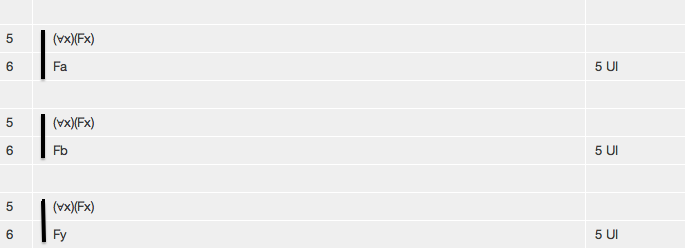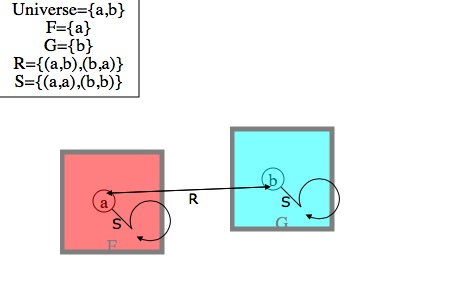# Tutorial 24: The restrictions on the quantificational rules

Logical System

### Skill to be acquired:

To understand the concepts of scope, free, bound, and free for.

### Why this is useful:

The new predicate rules of inference using quantifiers have restrictions on them which are expressed in terms of these concepts.

### The Tutorial

There is a rule of derivation-- Universal Instantiation-- which, roughly speaking, allows you to do the following... if a formula has a Universal Quantifier as its main connective you may remove the Universal Quantifier and have as a new line the body of the formula with a term substituted in for all free occurrences of the variable of quantification within the scope. For example,are all proof fragments illustrating Universal Instantiation.

There is a restriction on the rule, but to understand the restriction you need to understand the concepts which are used to express it.

Notice the form of a formula with a quantifier as its main connective. Examples of such formulas are (∀x)(Fx), (∃x)(Fx∧Gx), (∀x)(Fx⊃Gx). In each case there is the quantifier with its variable, enclosed within brackets, and this is followed by a formula known as the scope of the quantifier. In our examples, (Fx) is the scope of the first one, (Fx∧Gx) the scope of the second, and (Fx⊃Gx) the scope of the third.

More formally...

If <scope> is any well-formed formula then (∀<variable>)<scope> is the universal quantification of <scope> using <variable> and (∃<variable>)<scope> is the existential quantification of <scope> using <variable> . In each case the <scope> is the scope of the quantification.

The notion of the scope of a quantifier is important; and it is good practice to enclose the scope in brackets so as to display exactly what it is. For example, the scope of the formula (∀x)Fx⊃Gx is Fx not (Fx⊃Gx); the formula as a whole will be read as ((∀x)(Fx))⊃Gx; such a formula is better written as (∀x)(Fx)⊃Gx.

A quantifier binds all occurrences of its <variable> in its scope. An occurrence of a variable which is not bound is called free. So, in (∀x)(Fx)⊃Gx the occurrence of x immediately after F is bound whereas the occurrence of x immediately after G is free; but in the formula (∀x)(Fx⊃Gx) both occurrences are bound.

Substitutions are on occasions made of one term for free occurrences of another-- in Universal Instantiation, for example.The notation <wff>[<newterm>/<oldterm>] means the formula <wff> with <newterm> substituted for the free occurrences of <oldterm> throughout. So, for example, (Fx⊃Gx)[a/x] is (Fa⊃Ga).

There is an obstacle to successful substitution, and that is capturing. Say we have a formula (∀x)(∃y)Lxy ('everybody loves somebody', perhaps) and we start taking instantiations of the universal quantifier by removing it to obtain the scope (∃y)Lxy and substituting terms for the free x ; thus, (∃y)Lay ('a loves somebody') (∃y)Lby ('b loves somebody'), this is fine and all these instantiations follow from the original statement but if we try to substitute y for x we get (∃y)Lyy ('somebody loves themself') which does not follow; the occurrence of x within the scope of the y quantifier is not free for the substitution y; such a substitution leads to capturing.

Capturing motivates the following definitions. Any term occurs within itself. A variable x is free for term t in formula F iff there is no free occurrence of x in F within the scope of a quantifier whose variable of quantification occurs in t.

Sounds terrible, doesn't it? But suppose F is

(Gxy ∧-(∃y)(Lxy))⊃((∀z)((Rx∧Sz) ≡ (∃x)(Lxw))

and the variable x is our interest;

• there are three free occurrences of x,
• four occurrences of x in total;
• the first free occurrence of x is not within the scope of another quantifier so that occurrence of x rules out nothing;
• the second free occurrence of x is within the scope of a quantifier with y as its variable of quantification so that free occurrence of x means that x is not free for any term in which y occurs;
• the third free occurrence of x is within the scope of a quantifier with z as its variable of quantification so that free occurrence of x means that x is not free for any term in which z occurs;
• the fourth occurrence of x is not free and so contributes nothing to this discussion;

so, as examples, x is free for a in F, x is free for w in F, x is free for x in F, but x is not free for y in F, but x is not free for z in F.

The full rule of Universal Instantiation needs to take account of capturing.

#### The Rule of Universal Instantiation UI

If a derivation contains a line of the form

n (∀<variable>)<scope> <any justification>

then a line of the form

<<scope>[<term>/<variable>]> 'n UI'

may be added to the derivation, provided that <variable> is free for <term> in the <scope>.

#### The Rules of Universal Generalization UG and Existential Instantiation EI

are correct as originally stated.

#### The Rule of Existential Generalization EG

If a derivation contains a line of the form

n. <formula>[<term>/<variable>] <any justification>

then a line of the form

(∃<variable>)<formula> 'n EG'

may be added to the derivation, provided that <variable> is free for <term> in the <scope>. The <formula> of line n is the <scope> of the existentially generalized formula.

## Exercise to accompany Predicate Tutorial 14.

### Exercise 2 (of 4)

The rule Universal Generalization has the restriction on it that the variable of quantification must not be free in the assumptions of the line on which it is used. The reason for this is that otherwise derivation of some invalid arguments would be possible.

Let us consider two arguments with free variables in the standing assumptions

a) Fx ∴ (∀y)Fx

and look at this semantically. Is is possible for all the premises to be true and the conclusion false at one and the same time?

Take the interpretation shown in the drawing

Universe= { a,b }
F= { a }

we cannot discuss the truth of Fx until we value its free variable and since we want it to be true we would try Fx[a/x] {ask for its truth} but under this valuation (∀y)Fx[a/x] is true also {ask}.

We might now suspect that the argument is valid, and indeed we can derive it (try it). Move on now to

b) Fx ∴ (∀x)Fx

Notice this time that the variable of quantification occurs free in the assumptions.

Look at this semantically. Is is possible for all the premises to be true and the conclusion false at one and the same time?

Take the interpretation shown in the drawing

Universe= { a,b }
F= { a }

we cannot discuss the truth of Fx until we value its free variable and since we want it to be true we would try Fx[a/x] {ask for its truth} but under this valuation (∀x)Fx[a/x] is false {ask}.

This means that the argument is invalid! The interpretation andvaluation prove it to be so. (Try doing a derivation if you wish.)

Arguments with free variables in their standing assumptions are relatively rare. But the rule for Existential Instantiation nearly always introduces a free variable into the current assumptions, because you take its scope as a new assumption and this will nearly always contain the variable that was used with the Existential Quantifier. So, if you start an Existential Instantiation and then use Universal Generalization, be careful.

### Exercise 3(of 4)

The rule Existential Generalization has the restriction on it that no occurrence of the term that you are generalizing on is bound by another quantifier. In other words, working back from the result back substitution should not result in capturing (in the terminology... the variable of generalization must be free for the term of generalization in the scope).

So, for example,

(∀x)Sxa ∴ (∃y)(∀x)Sxy
(∀x)Sxb ∴ (∃y)(∀x)Sxy
(∀x)Sxy ∴ (∃y)(∀x)Sxy
(∀x)Sxz ∴ (∃y)(∀x)Sxy

are all valid arguments (and may be proved so by one use of Existential Generalization). Derive them.

But

(∀x)Sxx ∴ (∃y)(∀x)Sxy

is invalid. Look at the interpretation of the diagram and note that all the premises are true and the conclusion false at one and the same time. This argument cannot be proved to be valid (for it is not). But the unwary might think that they can prove it by one use of Existential Generalization which generalizes on one occurrence of x (but this is the forbidden case where the occurrence is already quantified by another quantifier-- back substituting the x leads to it getting captured by the other quantifier).

### Exercise 4(of 4)

The rule Existential Instantiation has on it two restrictions.

First the variable of instantiation must not occur free in the proposed target instantiation. Consider the following argument.

(∃x)Fx∴Fx

It is invalid. To see this we have to value the free x in the conclusion. Thus

(∃x)Fx∴Fx[b/x]

In the diagram, this has true premises and a false conclusion (check it). The first restriction on the EI rule stops invalid arguments, like the example, from being derived. Roughly speaking what is going on here is that the premise tells us at least one thing is F, and the conclusion tells us that x is F, but x is a free variable which might name anything in the Universe, and there are valuations in which at least one thing is F but x does not name any of them.

The second restriction on the EI rule is that the variable of instantiation must not occur free in the assumptions of the intended target.

Consider the argument.

Gx, (∃x)Fx∴(∃x)(Fx∧Gx)

It is invalid. To see this we have to value the free x in the premises. Thus

Gx[b/x], (∃x)Fx∴(∃x)(Fx∧Gx)

In the diagram, this has true premises and a false conclusion(check it).

The second restriction on the EI rule stops invalid arguments, like the example, from being derived. Roughly speaking what is going on here is that one premise tells us at least one thing is F, and the other premise tells us that x is G, but x is a free variable which might name anything in the Universe, and there are valuations in which at least one thing is F but the x which is G does not name any of them (sure there is an F, sure there is a G, but there is no guarantee that something is both F and G).

The Interpretation in Predex14 Ex4  shows an Interpretation similar to thisif it does not draw a similar one for yourself.

Interpretations Widget